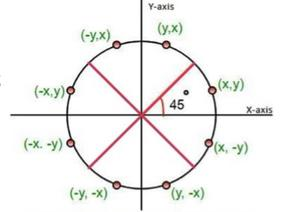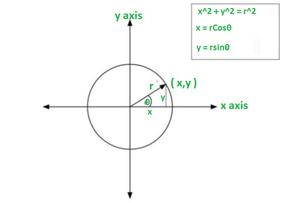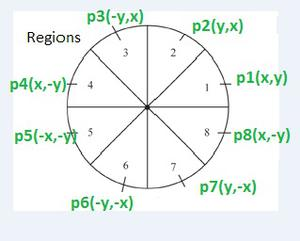# Scan conversion methods of circle and circle generation algorithms

• Difficulty Level : Expert
• Last Updated : 17 Jun, 2021

Scan conversion of circle :

• A circle is defined as a set of points that all are the same distance from a common point.
• The Centre of the circle is known as the Centre, and the radius is the distance from the Centre of the circle to any point on its circumference.
• It’s an eight-sided symmetrical figure divided into four quadrants. By knowing only one point and reflecting it via every 45 degree axis, this symmetry aids in constructing a circle on a computer.fig = scan conversion of circle

METHODS :
Method I – Direct or Polynomial Method :

• In this method, a circle is defined with the help of a polynomial equation.

Method II – Polar Coordinates Method :

• In this method, the coordinates are converted into polar coordinates.FIG = polar form

Circle generation algorithms :
1) Mid-Point Circle Algorithm :

• The mid-point circle drawing algorithm is used to calculate all the perimeter points of a circle.
• In this algorithm, the mid-point between the two pixels is calculated which helps in calculating the decision parameter.
• The value of the decision parameter will decide which pixel should be chosen for drawing the circle.
• This algorithm only calculates the points for one octant and the points for other octants are generated using the eight-way symmetry for the circle.

Algorithm :

• Step 1: Plot the initial point such that x = 0 and y = r.
• Step 2: Find initial decision parameter: P=5/4 – r.
• Step 3: if (P< 0): Set P= P+ 2x + 3 and yN = y , xN = x + 1 else if (P>= 0): Set P= P+ 2(x-y) + 5 and yN = y – 1 , xN = x + 1.
• Step 4: Plot the complete circle by using 8-way symmetry.FIG = Mid-point circle algorithm

• The Bresenham’s circle drawing algorithm is a circle drawing algorithm which calculates all the nearest points nearest to the circle boundary.
• It is an incremental method. •
• It only uses integer arithmetic which makes it’s working faster as well as less complex.
• The strategy that is followed in this algorithm is to select the pixel which has the least distance with the true circle boundary and with then keep calculating the successive points on the circle.
• As we know that the circle follows 8 symmetry property, i.e. if we know the boundary coordinates of the first octant, the rest 7 octants’ value can be easily calculated by changing their magnitudes or by interchanging the coordinate values according to the respective octants.
• This algorithm calculates the location of the pixels similarly.
• Here, we calculate the values only for the first octant and the values for the rest octants can be calculated by extending these values to the other 7 octants, using the eight-way symmetry property of the circle.

Algorithm :

• Step 1: Initialize x = 0 , y = r.
• Step 2: Calculate the decision parameter as follows: D = 3 – 2r.
• Step 3: if D < 0: then D = D + 4x + 6 xN = x + 1 yN = y if D ≥ 0: then D = D + 4 (x – y) + 10 xN = x + 1 yN = y – 1
• Step 4: Plot the complete circle by using 8-way symmetry .fig = Bresenham’s circle algorithm

My Personal Notes arrow_drop_up